## Demand Curves Perceived by a Perfectly Competitive Firm and by a Monopoly

Consider a monopoly firm, comfortably surrounded by barriers to entry so that it need not fear competition from other producers. How will this monopoly choose its profit-maximizing quantity of output, and what price will it charge? Profits for the monopolist, like any firm, will be equal to total revenues minus total costs. The pattern of costs for the monopoly can be analyzed within the same framework as the costs of a perfectly competitive firm—that is, by using total cost, fixed cost, variable cost, marginal cost, average cost, and average variable cost. However, because a monopoly faces no competition, its situation and its decision process will differ from that of a perfectly competitive firm.

A perfectly competitive firm acts as a price taker, so its calculation of total revenue is made by taking the given market price and multiplying it by the quantity of output that the firm chooses. The demand curve as it is perceived by a perfectly competitive firm appears in Figure 9.3 (a). The flat perceived demand curve means that, from the viewpoint of the perfectly competitive firm, it could sell either a relatively low quantity like Ql or a relatively high quantity like Qh at the market price P.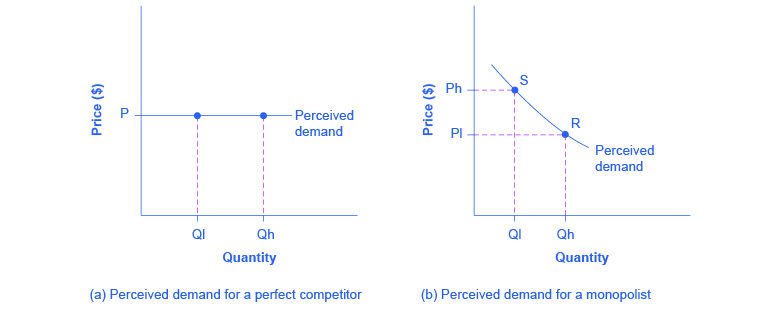Figure 9.3. The Perceived Demand Curve for a Perfect Competitor and a Monopolist. (a) A perfectly competitive firm perceives the demand curve that it faces to be flat. The flat shape means that the firm can sell either a low quantity (Ql) or a high quantity (Qh) at exactly the same price (P). (b) A monopolist perceives the demand curve that it faces to be the same as the market demand curve, which for most goods is downward-sloping. Thus, if the monopolist chooses a high level of output (Qh), it can charge only a relatively low price (Pl); conversely, if the monopolist chooses a low level of output (Ql), it can then charge a higher price (Ph). The challenge for the monopolist is to choose the combination of price and quantity that maximizes profits.

## What Defines the Market?

A monopoly is a firm that sells all or nearly all of the goods and services in a given market. But what defines the “market”?

In a famous 1947 case, the federal government accused the DuPont company of having a monopoly in the cellophane market, pointing out that DuPont produced 75% of the cellophane in the United States. DuPont countered that even though it had a 75% market share in cellophane, it had less than a 20% share of the “flexible packaging materials,” which includes all other moisture-proof papers, films, and foils. In 1956, after years of legal appeals, the U.S. Supreme Court held that the broader market definition was more appropriate, and the case against DuPont was dismissed.

Questions over how to define the market continue today. True, Microsoft in the 1990s had a dominant share of the software for computer operating systems, but in the total market for all computer software and services, including everything from games to scientific programs, the Microsoft share was only about 16% in 2000. The Greyhound bus company may have a near-monopoly on the market for intercity bus transportation, but it is only a small share of the market for intercity transportation if that market includes private cars, airplanes, and railroad service. DeBeers has a monopoly in diamonds, but it is a much smaller share of the total market for precious gemstones and an even smaller share of the total market for jewelry. A small town in the country may have only one gas station: is this gas station a “monopoly,” or does it compete with gas stations that might be five, 10, or 50 miles away?

In general, if a firm produces a product without close substitutes, then the firm can be considered a monopoly producer in a single market. But if buyers have a range of similar—even if not identical—options available from other firms, then the firm is not a monopoly. Still, arguments over whether substitutes are close or not close can be controversial.

While a monopolist can charge any price for its product, that price is nonetheless constrained by demand for the firm’s product. No monopolist, even one that is thoroughly protected by high barriers to entry, can require consumers to purchase its product. Because the monopolist is the only firm in the market, its demand curve is the same as the market demand curve, which is, unlike that for a perfectly competitive firm, downward-sloping.

Figure 9.3 illustrates this situation. The monopolist can either choose a point like R with a low price (Pl) and high quantity (Qh), or a point like S with a high price (Ph) and a low quantity (Ql), or some intermediate point. Setting the price too high will result in a low quantity sold, and will not bring in much revenue. Conversely, setting the price too low may result in a high quantity sold, but because of the low price, it will not bring in much revenue either. The challenge for the monopolist is to strike a profit-maximizing balance between the price it charges and the quantity that it sells. But why isn’t the perfectly competitive firm’s demand curve also the market demand curve?

## WHAT IS THE DIFFERENCE BETWEEN PERCEIVED DEMAND AND MARKET DEMAND?

The demand curve as perceived by a perfectly competitive firm is not the overall market demand curve for that product. However, the firm’s demand curve as perceived by a monopoly is the same as the market demand curve. The reason for the difference is that each perfectly competitive firm perceives the demand for its products in a market that includes many other firms; in effect, the demand curve perceived by a perfectly competitive firm is a tiny slice of the entire market demand curve. In contrast, a monopoly perceives demand for its product in a market where the monopoly is the only producer.

## Total Cost and Total Revenue for a Monopolist

Profits for a monopolist can be illustrated with a graph of total revenues and total costs, as shown with the example of the hypothetical HealthPill firm in Figure 9.4. The total cost curve has its typical shape; that is, total costs rise and the curve grows steeper as output increases.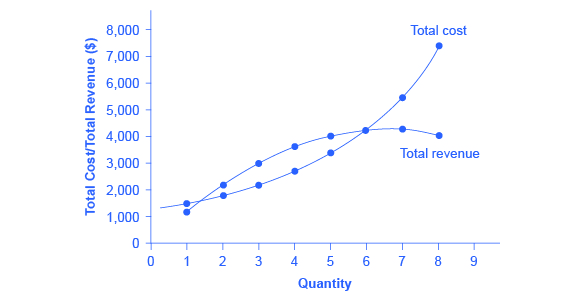Figure 9.4. Total Revenue and Total Cost for the HealthPill Monopoly Total revenue for the monopoly firm called HealthPill first rises, then falls. Low levels of output bring in relatively little total revenue, because the quantity is low. High levels of output bring in relatively less revenue, because the high quantity pushes down the market price. The total cost curve is upward-sloping. Profits will be highest at the quantity of output where total revenue is most above total cost. Of the choices in Table 9.2, the highest profits happen at an output of 4. The profit-maximizing level of output is not the same as the revenue-maximizing level of output, which should make sense, because profits take costs into account and revenues do not.

Table 9.2 Total Costs and Total Revenues of HealthPill
Quantity Total Cost Quantity Price Total Revenue Profit = Total Revenue – Total Cost
1 1,500 1 1,200 1,200 –300
2 1,800 2 1,100 2,200 400
3 2,200 3 1,000 3,000 800
4 2,800 4 900 3,600 900
5 3,500 5 800 4,000 700
6 4,200 6 700 4,200 0
7 5,600 7 600 4,200 –1,400
8 7,400 8 500 4,000 –3,400

To calculate total revenue for a monopolist, start with the demand curve perceived by the monopolist. Table 9.2 shows quantities along the demand curve and the price at each quantity demanded, and then calculates total revenue by multiplying price times quantity at each level of output. (In this example, the output is given as 1, 2, 3, 4, and so on, for the sake of simplicity. If you prefer a dash of greater realism, you can imagine that these output levels and the corresponding prices are measured per 1,000 or 10,000 pills.) As the figure illustrates, total revenue for a monopolist rises, flattens out, and then falls. In this example, total revenue is highest at a quantity of 6 or 7.

Clearly, the total revenue for a monopolist is not a straight upward-sloping line, in the way that total revenue was for a perfectly competitive firm. The different total revenue pattern for a monopolist occurs because the quantity that a monopolist chooses to produce affects the market price, which was not true for a perfectly competitive firm. If the monopolist charges a very high price, then quantity demanded drops, and so total revenue is very low. If the monopolist charges a very low price, then, even if quantity demanded is very high, total revenue will not add up to much. At some intermediate level, total revenue will be highest.

However, the monopolist is not seeking to maximize revenue, but instead to earn the highest possible profit. Profits are calculated in the final row of the table. In the HealthPill example in Figure 9.4, the highest profit will occur at the quantity where total revenue is the farthest above total cost. Of the choices given in the table, the highest profits occur at an output of 4, where profit is 900.

## Marginal Revenue and Marginal Cost for a Monopolist

In the real world, a monopolist often does not have enough information to analyze its entire total revenues or total costs curves; after all, the firm does not know exactly what would happen if it were to alter production dramatically. But a monopolist often has fairly reliable information about how changing output by small or moderate amounts will affect its marginal revenues and marginal costs, because it has had experience with such changes over time and because modest changes are easier to extrapolate from current experience. A monopolist can use information on marginal revenue and marginal cost to seek out the profit-maximizing combination of quantity and price.

The first four columns of Table 9.3 use the numbers on total cost from the HealthPill example in the previous exhibit and calculate marginal cost and average cost. This monopoly faces a typical U-shaped average cost curve and upward-sloping marginal cost curve, as shown in Figure 9.5. The second four columns of Table 9.3 use the total revenue information from the previous exhibit and calculate marginal revenue.

Notice that marginal revenue is zero at a quantity of 7, and turns negative at quantities higher than 7. It may seem counterintuitive that marginal revenue could ever be zero or negative: after all, does an increase in quantity sold not always mean more revenue? For a perfect competitor, each additional unit sold brought a positive marginal revenue, because marginal revenue was equal to the given market price. But a monopolist can sell a larger quantity and see a decline in total revenue. When a monopolist increases sales by one unit, it gains some marginal revenue from selling that extra unit, but also loses some marginal revenue because every other unit must now be sold at a lower price. As the quantity sold becomes higher, the drop in price affects a greater quantity of sales, eventually causing a situation where more sales cause marginal revenue to be negative.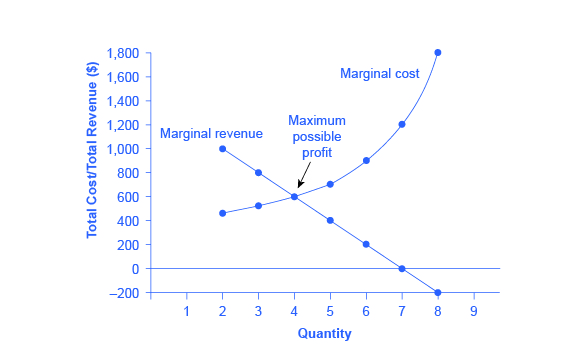Figure 9.5. Marginal Revenue and Marginal Cost for the HealthPill Monopoly. For a monopoly like HealthPill, marginal revenue decreases as additional units are sold. The marginal cost curve is upward-sloping. The profit-maximizing choice for the monopoly will be to produce at the quantity where marginal revenue is equal to marginal cost: that is, MR = MC. If the monopoly produces a lower quantity, then MR > MC at those levels of output, and the firm can make higher profits by expanding output. If the firm produces at a greater quantity, then MC > MR, and the firm can make higher profits by reducing its quantity of output.

Table 9.3 Costs and Revenues of HealthPill
Cost Information Revenue Information
Quantity Total Cost Marginal Cost Average Cost Quantity Price Total Revenue Total Revenue
1 1,500 1,500 1,500 1 1,200 1,200 1,200
2 1,800 300 900 2 1,100 2,200 1,000
3 2,200 400 733 3 1,000 3,000 800
4 2,800 600 700 4 900 3,600 600
5 3,500 700 700 5 800 4,000 400
6 4,400 900 733 6 700 4,200 200
7 5,600 1,200 800 7 600 4,200 0
8 7,400 1,800 925 8 500 4,000 –200

A monopolist can determine its profit-maximizing price and quantity by analyzing the marginal revenue and marginal costs of producing an extra unit. If the marginal revenue exceeds the marginal cost, then the firm should produce the extra unit.

For example, at an output of 3 in Figure 9.5, marginal revenue is 800 and marginal cost is 400, so producing this unit will clearly add to overall profits. At an output of 4, marginal revenue is 600 and marginal cost is 600, so producing this unit still means overall profits are unchanged. However, expanding output from 4 to 5 would involve a marginal revenue of 400 and a marginal cost of 700, so that fifth unit would actually reduce profits. Thus, the monopoly can tell from the marginal revenue and marginal cost that of the choices given in the table, the profit-maximizing level of output is 4.

Indeed, the monopoly could seek out the profit-maximizing level of output by increasing quantity by a small amount, calculating marginal revenue and marginal cost, and then either increasing output as long as marginal revenue exceeds marginal cost or reducing output if marginal cost exceeds marginal revenue. This process works without any need to calculate total revenue and total cost. Thus, a profit-maximizing monopoly should follow the rule of producing up to the quantity where marginal revenue is equal to marginal cost—that is, MR = MC.

If you find it counterintuitive that producing where marginal revenue equals marginal cost will maximize profits, working through the numbers will help.

Step 1. Remember that marginal cost is defined as the change in total cost from producing a small amount of additional output.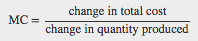Step 2. Note that in Table 9.3 , as output increases from 1 to 2 units, total cost increases from $1500 to$1800. As a result, the marginal cost of the second unit will be: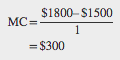Step 3. Remember that, similarly, marginal revenue is the change in total revenue from selling a small amount of additional output.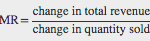Step 4. Note that in Table 9.3 , as output increases from 1 to 2 units, total revenue increases from $1200 to$2200. As a result, the marginal revenue of the second unit will be: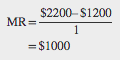Table 9.4 repeats the marginal cost and marginal revenue data from Table 9.3, and adds two more columns: Marginal profit is the profitability of each additional unit sold. It is defined as marginal revenue minus marginal cost. Finally, total profit is the sum of marginal profits. As long as marginal profit is positive, producing more output will increase total profits. When marginal profit turns negative, producing more output will decrease total profits. Total profit is maximized where marginal revenue equals marginal cost. In this example, maximum profit occurs at 4 units of output.

A perfectly competitive firm will also find its profit-maximizing level of output where MR = MC. The key difference with a perfectly competitive firm is that in the case of perfect competition, marginal revenue is equal to price (MR = P), while for a monopolist, marginal revenue is not equal to the price, because changes in quantity of output affect the price.

Table 9.4 Marginal Revenue, Marginal Cost, Marginal and Total Profit
Quantity Marginal Revenue Marginal Cost Marginal Profit Total Profit
1 1,200 1,500 –300 –300
2 1,000 300 700 400
3 800 400 400 800
4 600 600 0 800
5 400 700 –300 500
6 200 900 –700 –200
7 0 1,200 –1,200 –1,400